# RD Sharma Solutions for Class 6 Maths Chapter 19: Geometrical Constructions Exercise 19.4

The main aim of creating the following solutions is to make learning fun for the students. The fourth Exercise of Chapter 19 mainly consists of steps which are to be followed in the construction of angles by using a protractor. These steps are explained in a comprehensive manner to make them interesting for the students during their exam preparation. The solutions mainly improve logical thinking abilities among the students, which are essential from the exam point of view. To expertise in the subject and perform well in the exam, students can use RD Sharma Solutions Class 6 Maths Chapter 19 Geometrical Constructions Exercise 19.4 PDF which are available here.

## RD Sharma Solutions for Class 6 Maths Chapter 19: Geometrical Constructions Exercise 19.4 Download PDF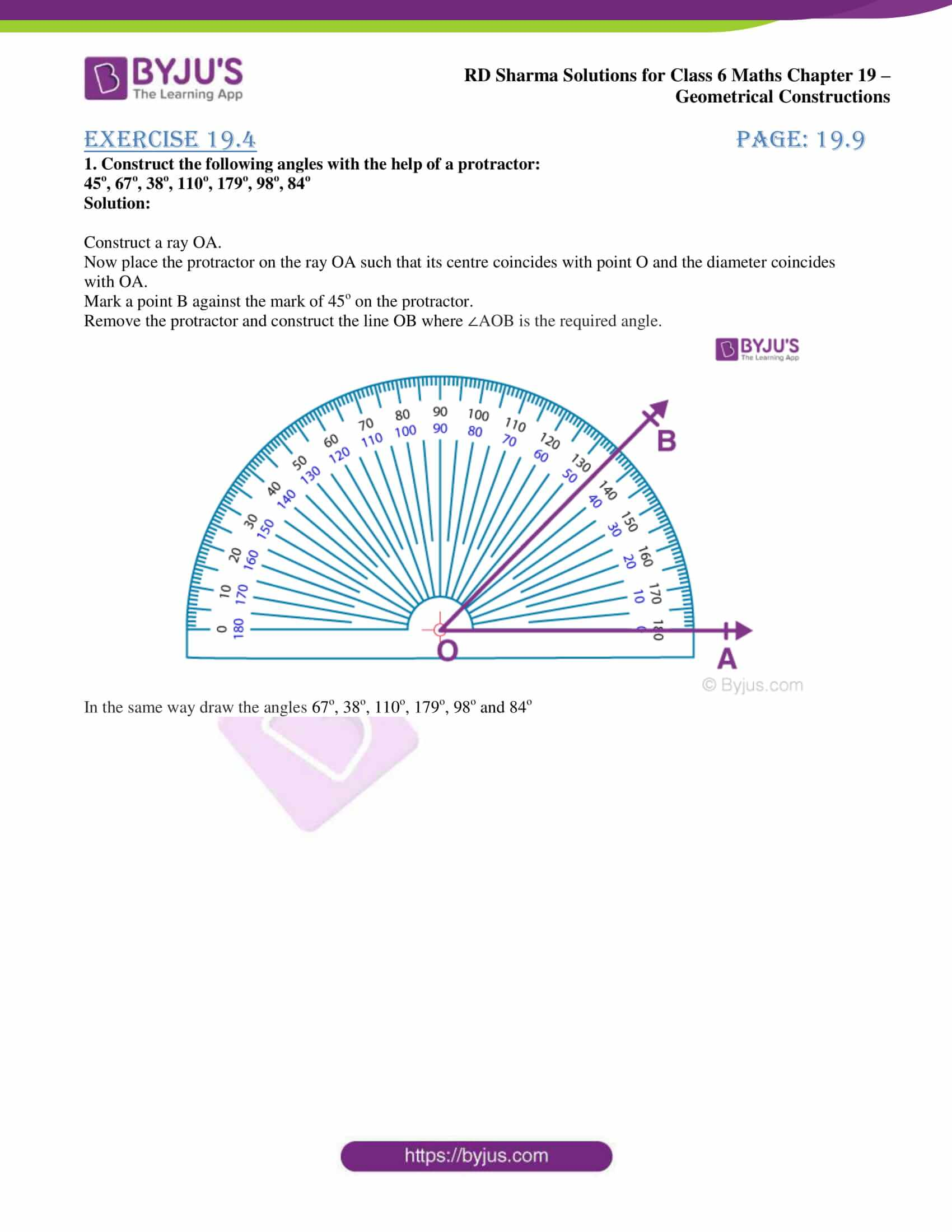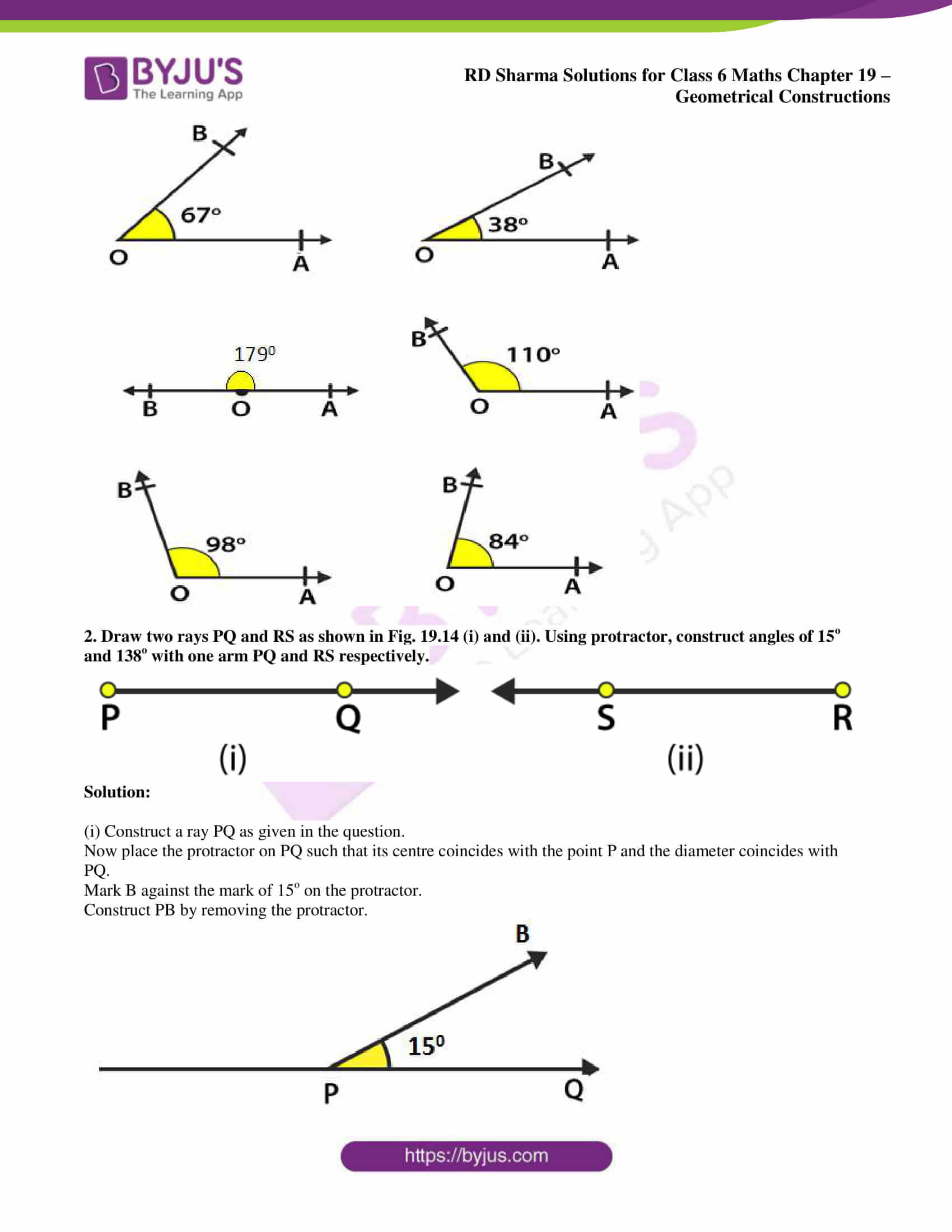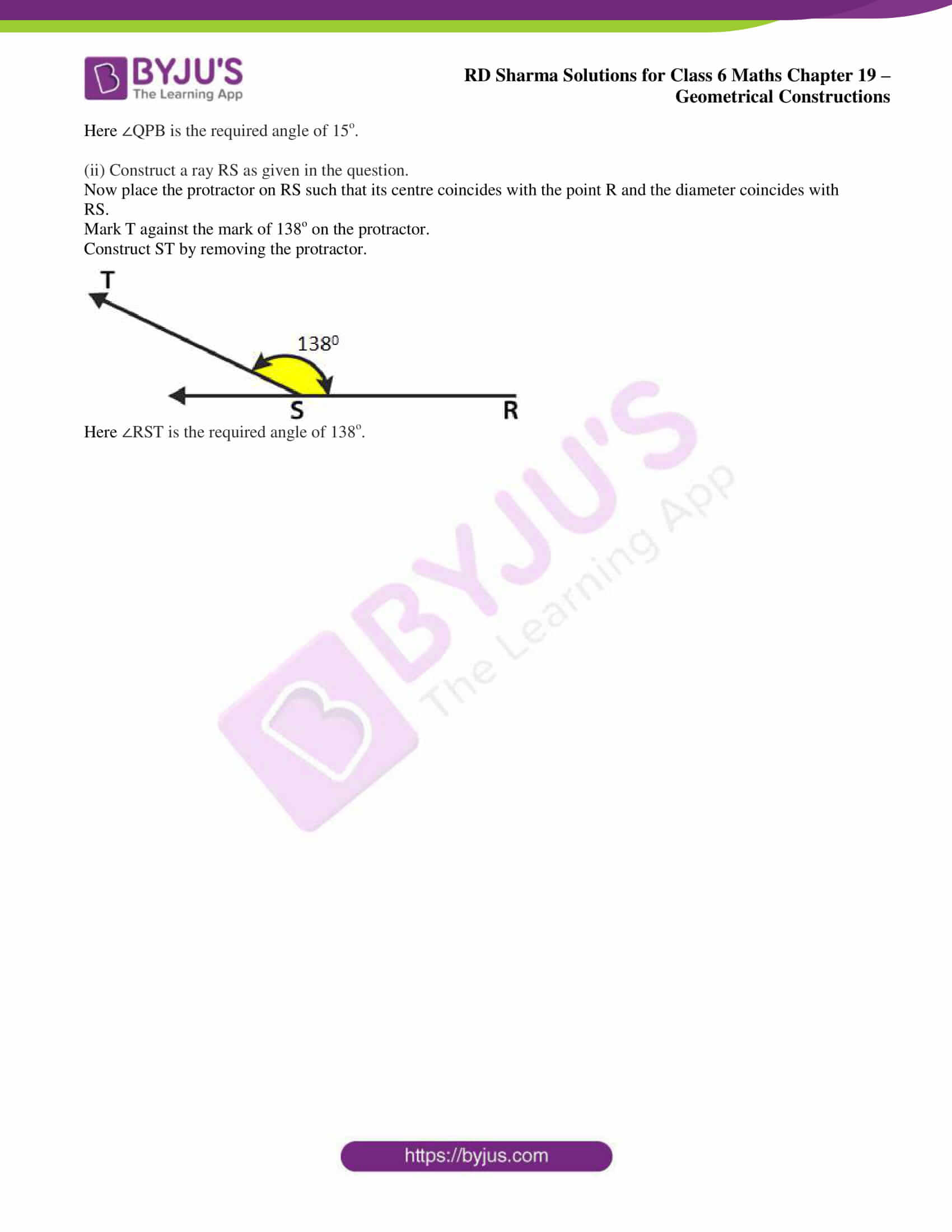### Exercise 19.4 page: 19.9

1. Construct the following angles with the help of a protractor:

45o, 67o, 38o, 110o, 179o, 98o, 84o

Solution:

Construct a ray OA.

Now place the protractor on the ray OA such that its centre coincides with point O and the diameter coincides with OA.

Mark a point B against the mark of 45o on the protractor.

Remove the protractor and construct the line OB where ∠AOB is the required angle.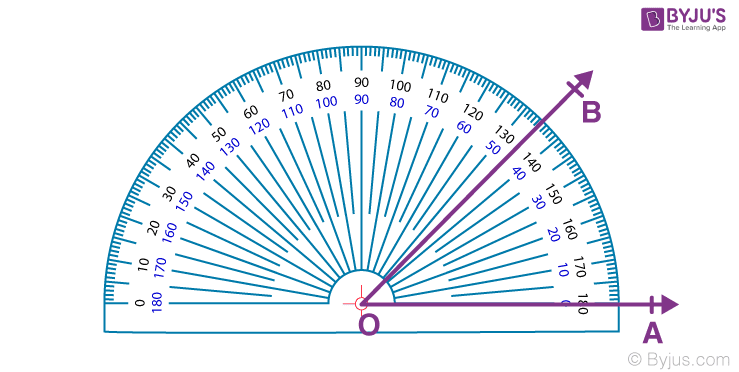In the same way draw the angles 67o, 38o, 110o, 179o, 98o and 84o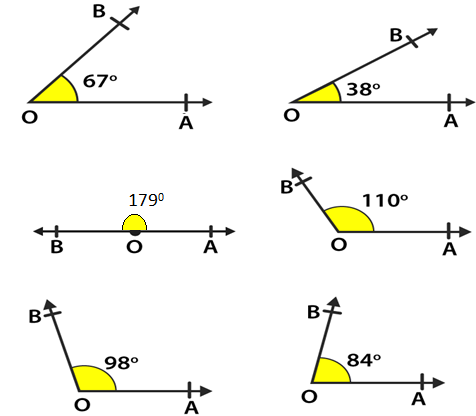2. Draw two rays PQ and RS as shown in Fig. 19.14 (i) and (ii). Using protractor, construct angles of 15o and 138o with one arm PQ and RS respectively.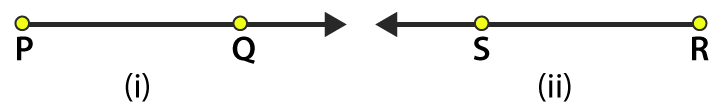Solution:

(i) Construct a ray PQ as given in the question.

Now place the protractor on PQ such that its centre coincides with the point P and the diameter coincides with PQ.

Mark B against the mark of 15o on the protractor.

Construct PB by removing the protractor.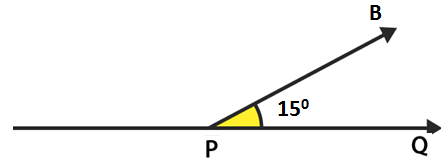Here ∠QPB is the required angle of 15o.

(ii) Construct a ray RS as given in the question.

Now place the protractor on RS such that its centre coincides with the point R and the diameter coincides with RS.

Mark T against the mark of 138o on the protractor.

Construct ST by removing the protractor.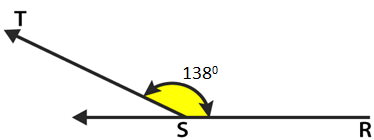Here ∠RST is the required angle of 138o.